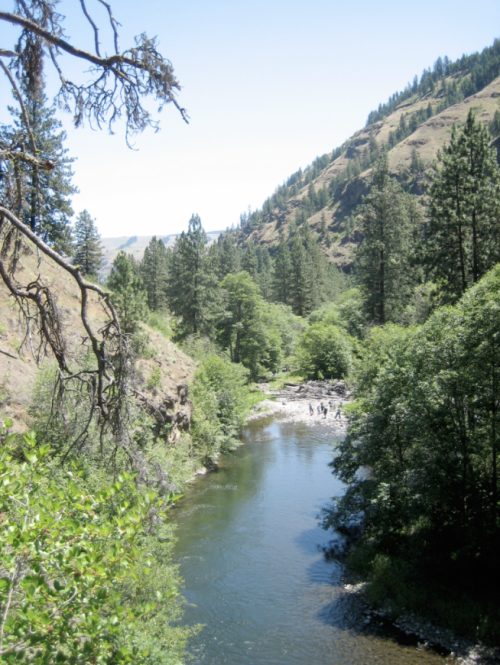# Reynolds Number and the Grande Ronde

Author
Ann Fissekis

The Reynolds number is a dimensionless number (i.e. it has no units) that describes whether a fluid has laminar or turbulent flow. It is a function of a fluid's density, kinematic viscosity, average velocity and the hydraulic diameter of the channel.

Re = ρDV/μ

Density and viscosity can be determined from reference tables; velocity and hydraulic diameters are measured. For this field log, the fluid being described is water.

Laminar flow describes sheets of water moving parallel to each other. Each sheet has a constant velocity, meaning all the water in that sheet moves at the same speed. Turbulent flow occurs when the different velocity layers bump into each other. Momentum is transferred from one water particle to another, causing a mix of velocity to occur in any one sheet.

One location where turbulence was detected was in a deep-flowing section of the Wenaha. Using a Marsh McBirney Flo-mate to measure velocity, multiple velocities were measured at one location approximately five meters from the left bank. Measurements taken at 6/10 depth near the deepest section of the channel showed velocities ranging from 0.43 m/s to .57 m/s. Because no average velocity could be detected, this implies that the water at this point was not moving in laminar sheets. Instead, the different layers of water were bumping into each other, causing the velocity to change erratically.Figure 1. Wenaha River pool (looking downstream) within which the velocity/depth profile was conducted.

Associated File(s)